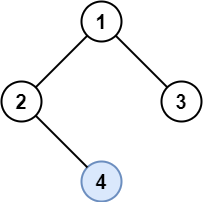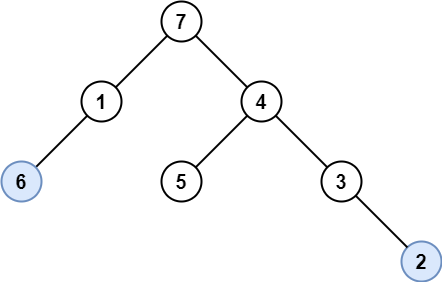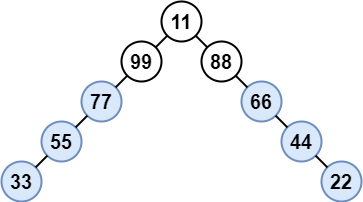Formatted question description: https://leetcode.ca/all/1469.html

# 1469. Find All the Lonely Nodes

Easy

## Description

In a binary tree, a lonely node is a node that is the only child of its parent node. The root of the tree is not lonely because it does not have a parent node.

Given the root of a binary tree, return an array containing the values of all lonely nodes in the tree. Return the list in any order.

Example 1:Input: root = [1,2,3,null,4]

Output: 

Explanation: Light blue node is the only lonely node.

Node 1 is the root and is not lonely.

Nodes 2 and 3 have the same parent and are not lonely.

Example 2:Input: root = [7,1,4,6,null,5,3,null,null,null,null,null,2]

Output: [6,2]

Explanation: Light blue nodes are lonely nodes. Please remember that order doesn’t matter, [2,6] is also an acceptable answer.

Example 3:Input: root = [11,99,88,77,null,null,66,55,null,null,44,33,null,null,22]

Output: [77,55,33,66,44,22]

Explanation: Nodes 99 and 88 share the same parent. Node 11 is the root. All other nodes are lonely.

Example 4:

Input: root = 

Output: []

Example 5:

Input: root = [31,null,78,null,28]

Output: [78,28]

Constraints:

• The number of nodes in the tree is in the range [1, 1000].
• Each node’s value is between [1, 10^6].

## Solution

Create a list to store the values of all lonely nodes. If root is null, return the list directly.

Do breadth first search. For each node, check its two children. For each non-empty child, offer the child to the queue for the next step’s search. If exactly one child is non-empty, then add the non-empty child’s value into the list.

Finally, return the list.

/**
* Definition for a binary tree node.
* public class TreeNode {
*     int val;
*     TreeNode left;
*     TreeNode right;
*     TreeNode() {}
*     TreeNode(int val) { this.val = val; }
*     TreeNode(int val, TreeNode left, TreeNode right) {
*         this.val = val;
*         this.left = left;
*         this.right = right;
*     }
* }
*/
class Solution {
public List<Integer> getLonelyNodes(TreeNode root) {
List<Integer> lonelyNodes = new ArrayList<Integer>();
if (root == null)
return lonelyNodes;
queue.offer(root);
while (!queue.isEmpty()) {
TreeNode node = queue.poll();
TreeNode left = node.left, right = node.right;
if (left != null && right != null) {
queue.offer(left);
queue.offer(right);
} else if (left != null) {
queue.offer(left);
} else if (right != null) {